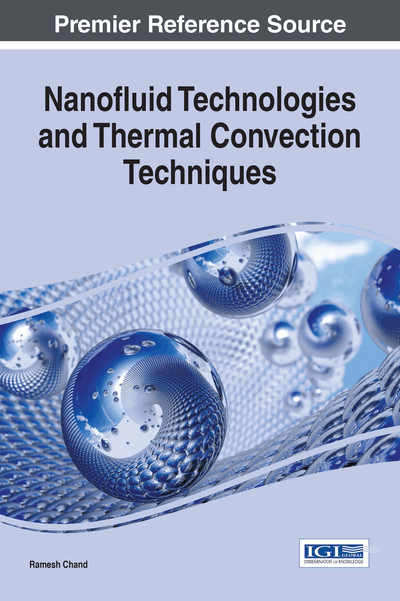# Thermal Convection in a Layer of Maxwellian Visco-Elastic Nanofluid in a Porous Medium: Darcy Model

Copyright: © 2017 |Pages: 20
DOI: 10.4018/978-1-68318-006-7.ch005

Top

## Mathematical Formulations Of The Problem

### The Physical Problem

In this chapter we shall investigate the thermal convection in a horizontal layer Maxwellian visco-elastic nanofluid. The physical configuration of the problem to be considered as:

An infinite horizontal layer of Maxwellian visco-elastic nanofluid of thickness‘d’ bounded by plane z = 0 and z = d, heated from below in a porous medium of medium permeability k1 and porosity ε. Fluid layer is acted upon by a gravity force g(0,0,-g) and is heated from below in such a way that horizontal boundaries z = 0 and z = d respectively maintained at a uniform temperature T0 and T1 (T0 > T1) as shown is Figure 1. The normal component of the nanoparticles flux has to vanish at an impermeable boundaries and the reference scale for temperature and nanoparticles fraction is taken to be T1 and φ0 respectively. It is assumed that nanoparticles are suspended in the nanofluid using either surfactant or surface charge technology. This prevents the particles from agglomeration and deposition on the porous matrix.

## Complete Chapter List

Search this Book:
Reset